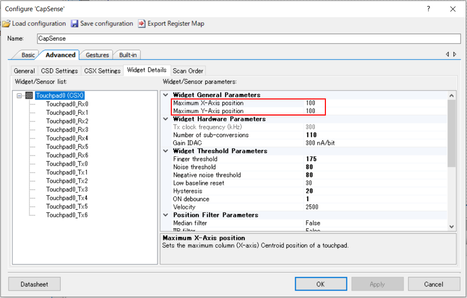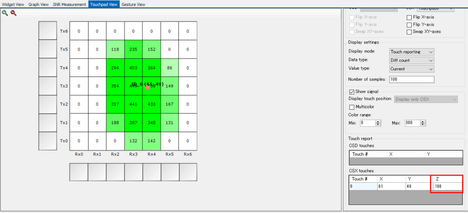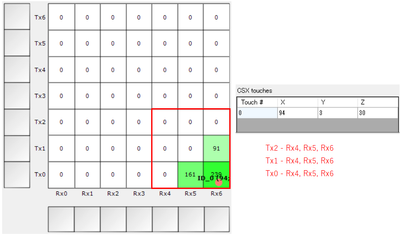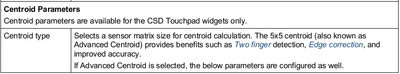# PSoC™ 4 Forum DiscussionsLevel 5
Level 5# TouchPad Z position in CSX of CE224821 with CY8CKIT041-40XX

Hello,

I am trying CE224821 CSX project with CY8CKIT041-40XX.

I have a question about the Z axis.

For the X and Y axes, the maximum X or Y axis position are configured.The z-axis seems to get bigger towards the center of the touchpad, but how is it defined and what is the maximum?Best regards,

Yocchi

1 SolutionModeratorModerator# Re: TouchPad Z position in CSX of CE224821 with CY8CKIT041-40XX

1. What is the "totalSum is the sum of centroid signal calculated across the 3x3 matrix" used for?

The total sum or the Z value is an indication of how big the finger press is or how strong the press is on the touchpad. This can be used for further processing such as small finger rejection.

2. Should the 3X3 matrix for the TX0-RX6 at the corner be as follows?z=(0+0+0+0+0+91+0+161+239)/16=30

Yes, this is correct. The center of the matrix will be the node with the highest signal. Your calculations are correct.

3. The Centroid parameter seems to be available only for CSD as shown below, but why does CSX use the 3x3 Centroid parameter for z-axis calculations?The documentation implies that you have the option to choose a 3x3 or a 5x5 matrix in the case of CSD. Therefore, the Centroid type is a configurable parameter for CSD. However, in CSX the centroid type is only 3x3 and not a configurable type.

Best regards,
Hari

3 RepliesModeratorModerator# Re: TouchPad Z position in CSX of CE224821 with CY8CKIT041-40XX

The Z value in touchpad is the amplitude of the touch signal checked across the 3x3 centroid. The z value is a sum of raw counts of sensors that form 3x3 matrix with a local maximum in the center. It is calculated as follows -

Where totalSum is the sum of centroid signal calculated across the 3x3 matrix.

Best regards,
HariLevel 5
Level 5# Re: TouchPad Z position in CSX of CE224821 with CY8CKIT041-40XX

Hello Hari,

I have three questions.

1. What is the "totalSum is the sum of centroid signal calculated across the 3x3 matrix" used for?

2. Should the 3X3 matrix for the TX0-RX6 at the corner be as follows?z=(0+0+0+0+0+91+0+161+239)/16=30

3. The Centroid parameter seems to be available only for CSD as shown below, but why does CSX use the 3x3 Centroid parameter for z-axis calculations?Best regards,

YocchiModeratorModerator# Re: TouchPad Z position in CSX of CE224821 with CY8CKIT041-40XX

1. What is the "totalSum is the sum of centroid signal calculated across the 3x3 matrix" used for?

The total sum or the Z value is an indication of how big the finger press is or how strong the press is on the touchpad. This can be used for further processing such as small finger rejection.

2. Should the 3X3 matrix for the TX0-RX6 at the corner be as follows?z=(0+0+0+0+0+91+0+161+239)/16=30

Yes, this is correct. The center of the matrix will be the node with the highest signal. Your calculations are correct.

3. The Centroid parameter seems to be available only for CSD as shown below, but why does CSX use the 3x3 Centroid parameter for z-axis calculations?The documentation implies that you have the option to choose a 3x3 or a 5x5 matrix in the case of CSD. Therefore, the Centroid type is a configurable parameter for CSD. However, in CSX the centroid type is only 3x3 and not a configurable type.

Best regards,
Hari